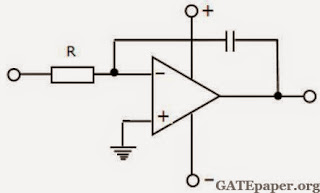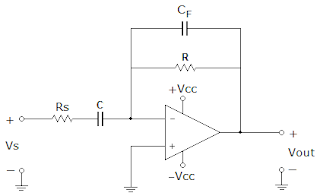### GATE 1991 ECE Analog Circuits (Analog Electronics) - Video Solutions

1. In figure, both transistors are identical and have a high value of β. Take the DC base emitter voltage drop as 0.7 volts and KT/q = 25 mV. The small signal low frequency voltage gain (Vo/Vi) is equal to __________2. In order that the circuit of figure works properly as differentiator it should be modified to __________(draw the modified circuit)3. Two non-inverting amplifiers, one having a unity gain and the other having a gain of 20 are made using identical operational amplifiers. As compared to unity gain amplifier, the amplifier with gain 20 has
a. Less negative feedback
b. Greater negative feedback
c. Less bandwidth
d. None of the above

4. In the figure, the operational amplifier is ideal and its output can swing between – 15 and + 15 volts.The input Vi, which is zero for t<0, is switched to 5 volts at the instant t = 0. Given that the output Vo is + 15 volts for t<0, sketch the waveforms of Vo and Vi. You must give the values of important parameters of the sketch.
Answer: Vo(t) = - 5t x 103 + 15

5. In figure, the operational amplifier are ideal and their output can swing between – 15 and + 15 volts.